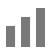# Defining calculation fields

Use calculation fields to perform calculations on the following types of data: text, number, date, time, or container.

The data in a calculation field is the result of a formula that you specify. The result can be one of these types of data: text, number, date, time, or container.

## To define calculation fields:

1. Choose File menu > Manage > Database.
2. Click the Fields tab.
3. If your file contains more than one table, select the appropriate table from the Table list.
4. For Field Name, type a name for the field.
5. For Type, select Calculation.
6. Click Create.

You see the Specify Calculation dialog box.

7. If necessary, for Evaluate this calculation from the context of, choose a table from the list.

Setting the context for a calculation is only necessary when you're creating a calculation field in a source table that has two or more occurrences in the relationships graph. The choice you make may affect the calculation results, particularly if your calculation will include fields in related tables. See Choosing the evaluation context for a calculation field.

8. Build a formula for your calculation using field references, operators, constant values, and functions. In the calculation pane, click where you want the item to appear, then:
9.  To add Do this A reference to a field Do one of the following: Begin typing the field's table, choose the table from the list, begin typing the field name, then choose the field name from the list that appears. In the fields pane, double-click the field name. To display field names from a different table, choose the table from the tables list. An operator or an exponent Do one of the following: Type an operator. Click an operator in the list to the right of the calculation pane. A constant value Type the value. A function Do one of the following: Begin typing the function name, then choose the function name from the list that appears. In the functions pane, double-click the function. In the calculation pane, replace the placeholder parameter with a value or expression.
10. Specify calculation options for the field.
11.  To Do this Set the field type of the result Choose a data type for Calculation result is . Choose the correct type for the result you want. See About choosing a field type. Make a calculated field repeating For Number of repetitions, type the number of repetitions. See Defining repeating fields. Prevent calculation if all referenced fields are empty Select Do not evaluate if all referenced fields are empty.
12. To select indexing and storage options for the field, click Storage Options, choose options in the Storage Options dialog box, then click OK.
13. Click OK, then click OK.

## Notes

• To filter the list of fields or functions in the fields pane or the functions pane, type the field name or function name in the search box.
• To change the way fields or functions are displayed in the fields pane or the functions pane, click• To produce a calculated result using values in more than one record in the database, see Defining summary fields.
• Calculation fields are updated when a value in the calculation changes. Unstored calculation fields are also updated when the record is refreshed, the field is clicked or tabbed into or out of, or the field is refreshed (for example, by a script).
• Values, expressions, functions, and parameters can be uppercase or lowercase.
• To include literal quotation marks in a calculation text string, precede the quotation mark character with a backslash character (\). For, example, `"Hello"` included in a calculation evaluates to `Hello`, but `"\"Hello\"" `evaluates to `"Hello".`
• Calculations that include a related field, summary field, global value, or a reference to another unstored calculation are unstored.
• Calculations defined with global storage options display values calculated using the last modified record.
• If any field on a layout containing unstored calculations is set to auto-resize, calculation results will be updated when the window is resized.
• Results in stored calculation fields are recalculated when user input or script actions modify fields that are referenced in the calculation. Results are updated in the order the calculation fields were created.

## Calculation examples

 Field name Field type Calculation Today's Date Date `Get( CurrentDate )` Full Name Text `FirstName & " " & LastName` Sales Tax Number `SubTotal * .08`